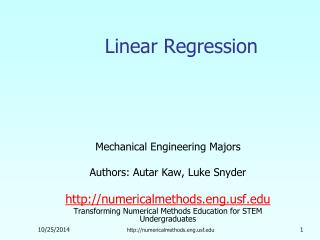# Linear Regression - PowerPoint PPT PresentationDownload PresentationLinear Regression

Presentation Description
Download Presentation## Linear Regression

- - - - - - - - - - - - - - - - - - - - - - - - - - - E N D - - - - - - - - - - - - - - - - - - - - - - - - - - -
##### Presentation Transcript

1. Linear Regression Mechanical Engineering Majors Authors: Autar Kaw, Luke Snyder http://numericalmethods.eng.usf.edu Transforming Numerical Methods Education for STEM Undergraduates http://numericalmethods.eng.usf.edu

2. Linear Regressionhttp://numericalmethods.eng.usf.edu

3. What is Regression? What is regression?Given n data points best fit to the data. The best fit is generally based on minimizing the sum of the square of the residuals, . Residual at a point is Sum of the square of the residuals Figure. Basic model for regression http://numericalmethods.eng.usf.edu

4. y x Linear Regression-Criterion#1 Given n data points best fit to the data. Figure. Linear regression of y vs. x data showing residuals at a typical point, xi . Does minimizing work as a criterion, where http://numericalmethods.eng.usf.edu

5. Example for Criterion#1 Example: Given the data points (2,4), (3,6), (2,6) and (3,8), best fit the data to a straight line using Criterion#1 Table. Data Points Figure. Data points for y vs. x data. http://numericalmethods.eng.usf.edu

6. Linear Regression-Criteria#1 Using y=4x-4 as the regression curve Table. Residuals at each point for regression model y = 4x – 4. Figure. Regression curve for y=4x-4, y vs. x data http://numericalmethods.eng.usf.edu

7. Linear Regression-Criteria#1 Using y=6 as a regression curve Table. Residuals at each point for y=6 Figure. Regression curve for y=6, y vs. x data http://numericalmethods.eng.usf.edu

8. Linear Regression – Criterion #1 for both regression models of y=4x-4 and y=6. The sum of the residuals is as small as possible, that is zero, but the regression model is not unique. Hence the above criterion of minimizing the sum of the residuals is a bad criterion. http://numericalmethods.eng.usf.edu

9. y x Linear Regression-Criterion#2 Will minimizing work any better? Figure. Linear regression of y vs. x data showing residuals at a typical point, xi . http://numericalmethods.eng.usf.edu

10. Linear Regression-Criteria 2 Using y=4x-4 as the regression curve Table. The absolute residuals employing the y=4x-4 regression model Figure. Regression curve for y=4x-4, y vs. x data http://numericalmethods.eng.usf.edu

11. Linear Regression-Criteria#2 Using y=6 as a regression curve Table. Absolute residuals employing the y=6 model Figure. Regression curve for y=6, y vs. x data http://numericalmethods.eng.usf.edu

12. Linear Regression-Criterion#2 for both regression models of y=4x-4 and y=6. The sum of the errors has been made as small as possible, that is 4, but the regression model is not unique. Hence the above criterion of minimizing the sum of the absolute value of the residuals is also a bad criterion. Can you find a regression line for which and has unique regression coefficients? http://numericalmethods.eng.usf.edu

13. y x Least Squares Criterion The least squares criterion minimizes the sum of the square of the residuals in the model, and also produces a unique line. Figure. Linear regression of y vs. x data showing residuals at a typical point, xi . http://numericalmethods.eng.usf.edu

14. Finding Constants of Linear Model Minimize the sum of the square of the residuals: To find and we minimize with respect to and . giving http://numericalmethods.eng.usf.edu

15. Finding Constants of Linear Model Solving for and directly yields, and http://numericalmethods.eng.usf.edu

16. Example 1 The coefficient of thermal expansion of steel is given at discrete values of temperature, as shown in the table. If the data is regressed to a first order polynomial, Find the constants of the model. Table. Data points for thermal expansion vs. temperature http://numericalmethods.eng.usf.edu

17. Example 1 cont. The necessary summations are calculated as http://numericalmethods.eng.usf.edu

18. Example 1 cont. We can now calculate the value of using http://numericalmethods.eng.usf.edu

19. Example 1 cont. The value for can be calculated using where The regression model is now given by http://numericalmethods.eng.usf.edu

20. Example 1 cont. Question: Can you find the decrease in the diameter of a solid cylinder of radius 12” if the cylinder is cooled from a room temperature of 80°F to a dry-ice/alcohol bath with temperatures of −108°F? What would be the error if you used the thermal expansion coefficient at room temperature to find the answer? Figure. Linear regression of Coefficient of Thermal expansion vs. Temperature data http://numericalmethods.eng.usf.edu

21. Example 2 To find the longitudinal modulus of composite, the following data is collected. Find the longitudinal modulus, using the regression model Table. Stress vs. Strain data and the sum of the square of the residuals. Figure. Data points for Stress vs. Strain data http://numericalmethods.eng.usf.edu

22. Example 2 cont. Residual at each point is given by The sum of the square of the residuals then is Differentiate with respect to Therefore http://numericalmethods.eng.usf.edu

23. Example 2 cont. Table. Summation data for regression model With and Using http://numericalmethods.eng.usf.edu

24. Example 2 Results The equation describes the data. Figure. Linear regression for Stress vs. Strain data http://numericalmethods.eng.usf.edu

25. Additional Resources For all resources on this topic such as digital audiovisual lectures, primers, textbook chapters, multiple-choice tests, worksheets in MATLAB, MATHEMATICA, MathCad and MAPLE, blogs, related physical problems, please visit http://numericalmethods.eng.usf.edu/topics/linear_regression.html

26. THE END http://numericalmethods.eng.usf.edu